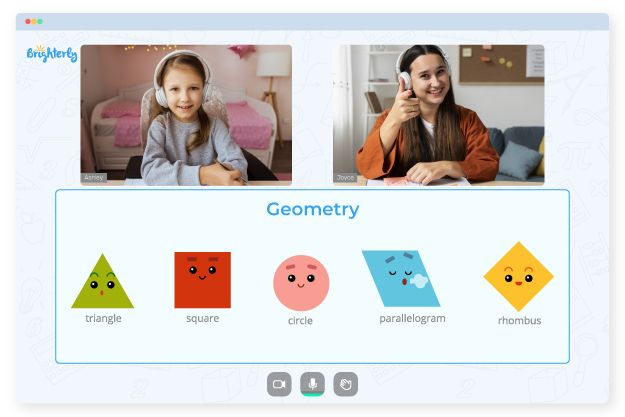# Area And Perimeter Worksheets Grade 3

Both area and perimeter are crucial mathematical concepts. An area refers to the space inside of things. To calculate the perimeter, you need to know how far something is from its edge. Area and perimeter are two aspects of geometry that are very important in numerous jobs, including artists, construction workers, engineers, etc. So, the two concepts are necessary for every third grader to learn. To make the process easier, here is how the area and perimeter worksheets grade 3 can help.

## How Do Brighterly Tutors Use Area and Perimeter Worksheets Grade 3

Brighterly is an online learning platform with professional tutors ready to teach your kid math with the aid of third grade area and perimeter worksheets grade 3 and games. They help kids understand that an area is a horizontal space occupied by a plane surface of a given form. Also, these professionals introduce kids to 3rd grade area and perimeter worksheets that allow students to know when to use a specific unit of measurements like square feet and square centimeters.

Math for Kids

Is Your Child Struggling With Math?
1:1 Online Math Tutoring## Studying with Perimeter and Area Worksheets 3rd Grade Is Fun!

Area and perimeter worksheets 3rd grade is fun as kids learn with color and exciting math activities. With these area and perimeter 3rd grade worksheets, you can be sure that kids will enjoy their calculations and solving process. Students can practice all they have learned and not only focus on the theoretical aspects.### Area And Perimeter Worksheets Grade 3 PDF

3Rd Grade Area And Perimeter Worksheets### Area And Perimeter Worksheets Grade 3 PDF### Area And Perimeter Worksheets Grade 3 PDF

Area And Perimeter Worksheets 3Rd Grade### Area And Perimeter Worksheets Grade 3 PDF

Area And Perimeter 3Rd Grade Worksheets

### More Geometry Worksheets

Need help with Geometry?• Does your child struggle with understanding geometry?
• An online tutor could be the answer.

Is your child having trouble grasping the concept of geometry? Try learning with an online tutor.